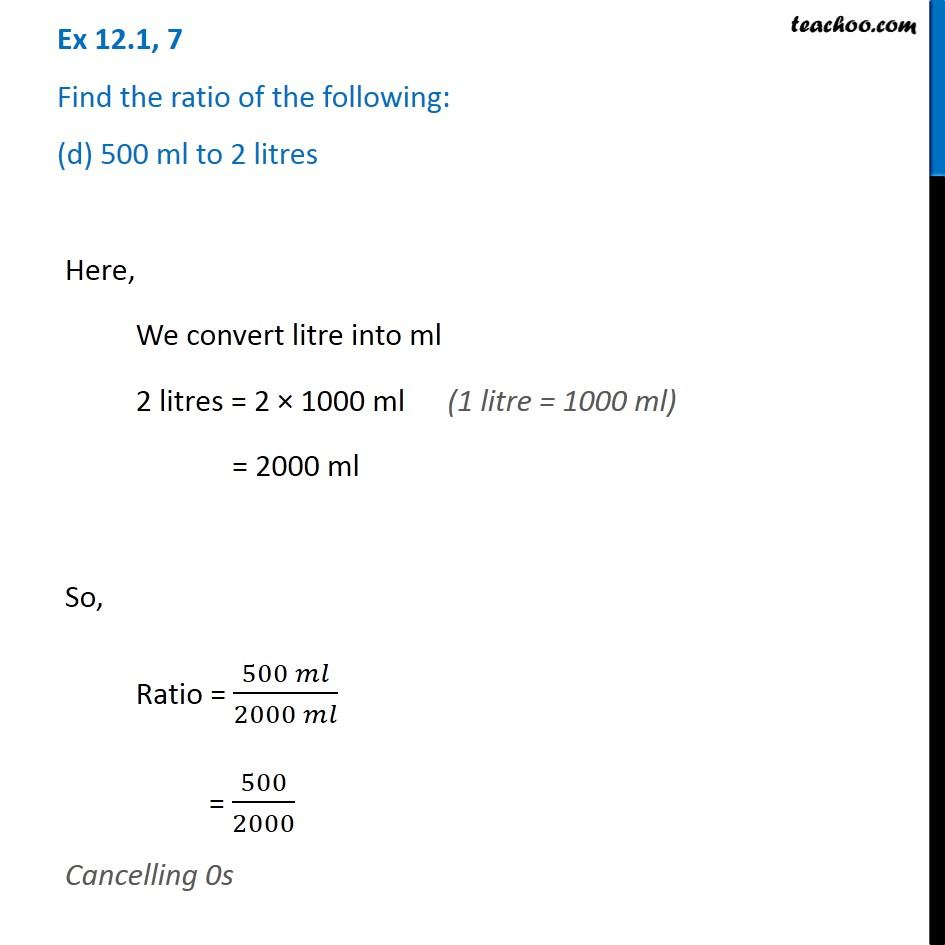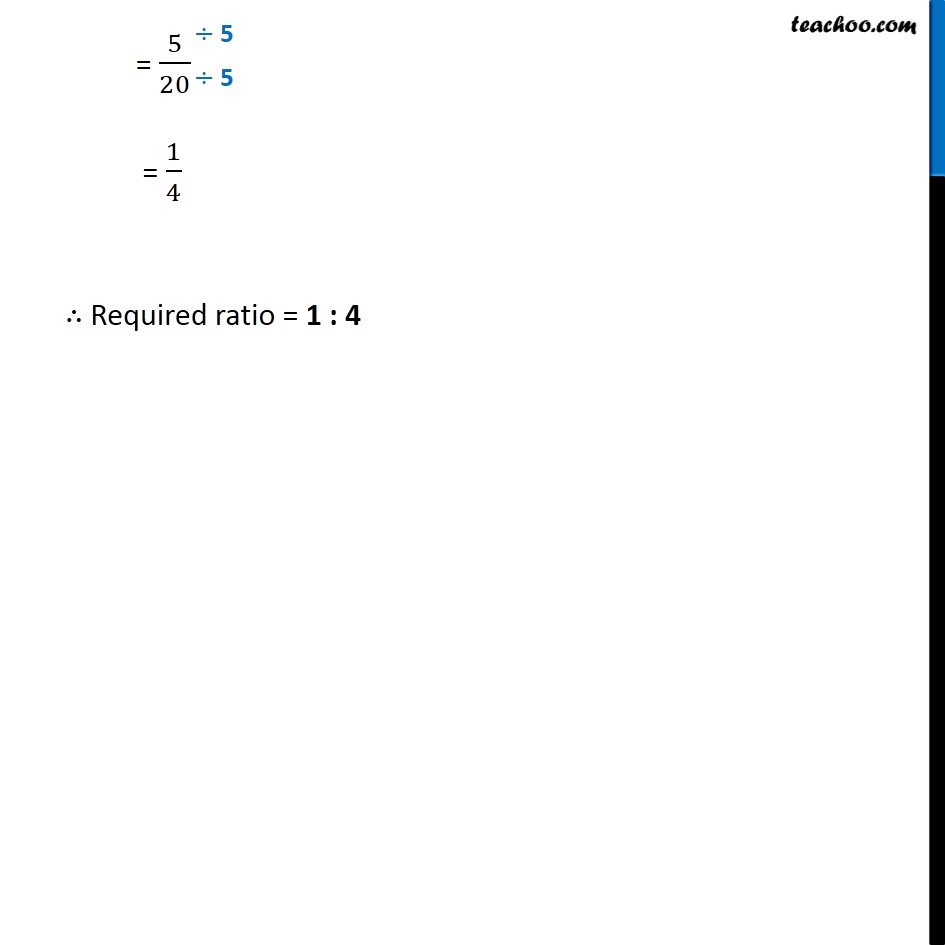Ex 12.1

Chapter 12 Class 6 Ratio and Proportion
Serial order wiseLearn in your speed, with individual attention - Teachoo Maths 1-on-1 Class

### Transcript

Ex 12.1, 7 Find the ratio of the following: (d) 500 ml to 2 litresHere, We convert litre into ml 2 litres = 2 × 1000 ml = 2000 ml So, Ratio = (500 𝑚𝑙)/(2000 𝑚𝑙) = 500/2000 Cancelling 0s = 5/20 = 1/4 ∴ Required ratio = 1 : 4